• 关于绘图库Matplotlib的安装方法：点击这里小编之前也和大家分享过python使用matplotlib实现的折线图和制饼图效果，感兴趣的朋友们也可以点击查看，下面来看看python使用matplotlib绘制柱状图的方法吧，具体如下：1....
Matplotlib的概念这里就不多介绍了，关于绘图库Matplotlib的安装方法：点击这里小编之前也和大家分享过python使用matplotlib实现的折线图和制饼图效果，感兴趣的朋友们也可以点击查看，下面来看看python使用matplotlib绘制柱状图的方法吧，具体如下：1. 基本的柱状图import matplotlib.pyplot as pltdata = [5, 20, 15, 25, 10]plt.bar(range(len(data)), data)plt.show()plt.bar函数签名为：bar(left, height, width=0.8, bottom=None, **kwargs)事实上，left，height，width，bottom这四个参数确定了柱体的位置和大小。默认情况下，left为柱体的居中位置（可以通过align参数来改变left值的含义），即：(left - width / 2, bottom)为左下角位置(left + width / 2, bottom + height)为右上角位置例如：import matplotlib.pyplot as pltdata = [5, 20, 15, 25, 10]plt.bar([0.3, 1.7, 4, 6, 7], data, width=0.6, bottom=[10, 0, 5, 0, 5])plt.show()2. 设置柱体样式（1）颜色通过facecolor(或fc)关键字参数可以设置柱体颜色，例如：import matplotlib.pyplot as pltdata = [5, 20, 15, 25, 10]plt.bar(range(len(data)), data, fc='g')plt.show()通过color关键字参数 可以一次性设置多个颜色，例如：import matplotlib.pyplot as pltdata = [5, 20, 15, 25, 10]plt.bar(range(len(data)), data, color='rgb') # or color=['r', 'g', 'b']plt.show()（2）描边相关的关键字参数为：edgecolor 或 eclinestyle 或 lslinewidth 或 lw例如：import matplotlib.pyplot as pltdata = [5, 20, 15, 25, 10]plt.bar(range(len(data)), data, ec='r', ls='--', lw=2)plt.show()（3）填充hatch关键字可用来设置填充样式，可取值为：/, \, |, -, +, x, o, O, ., *。例如：import matplotlib.pyplot as pltdata = [5, 20, 15, 25, 10]plt.bar(range(len(data)), data, ec='k', lw=1, hatch='o')plt.show()3. 设置tick labelimport matplotlib.pyplot as pltdata = [5, 20, 15, 25, 10]labels = ['Tom', 'Dick', 'Harry', 'Slim', 'Jim']plt.bar(range(len(data)), data, tick_label=labels)plt.show()4. 堆叠柱状图通过bottom参数，可以绘制堆叠柱状图。例如：import numpy as npimport matplotlib.pyplot as pltsize = 5x = np.arange(size)a = np.random.random(size)b = np.random.random(size)plt.bar(x, a, label='a')plt.bar(x, b, bottom=a, label='b')plt.legend()plt.show()5. 并列柱状图绘制并列柱状图与堆叠柱状图类似，都是绘制多组柱体，只需要控制好每组柱体的位置和大小即可。例如：import numpy as npimport matplotlib.pyplot as pltsize = 5x = np.arange(size)a = np.random.random(size)b = np.random.random(size)c = np.random.random(size)total_width, n = 0.8, 3width = total_width / nx = x - (total_width - width) / 2plt.bar(x, a, width=width, label='a')plt.bar(x + width, b, width=width, label='b')plt.bar(x + 2 * width, c, width=width, label='c')plt.legend()plt.show()6. 条形图使用barh方法绘制条形图。例如：import matplotlib.pyplot as pltdata = [5, 20, 15, 25, 10]plt.barh(range(len(data)), data)plt.show()plt.barh方法的签名为：barh(bottom, width, height=0.8, left=None, **kwargs)可以看到与plt.bar方法类似。因此堆积条形图和并列条形图的画法与前面类似，不做赘述。7. 正负条形图import numpy as npimport matplotlib.pyplot as plta = np.array([5, 20, 15, 25, 10])b = np.array([10, 15, 20, 15, 5])plt.barh(range(len(a)), a)plt.barh(range(len(b)), -b)plt.show()总结以上就是这篇文章的全部内容了，希望本文的内容对大家学习或者使用python能带来一定的帮助，如果有疑问大家可以留言交流。
展开全文• 目录1 问题2 从柱状图看绘制完整统计图注意事项3 Python3代码4 参考文献1 问题 同样是统计图，有的美观的表达了想法，有的却让人看不明白。那么，我们在绘制完整统计图时要注意哪些事项呢？下面通过柱状图来说明。2 ...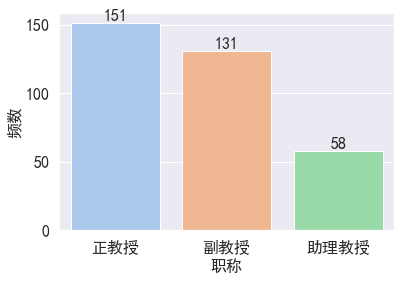目录1     问题2    从柱状图看绘制完整统计图注意事项3    Python3代码4    参考文献1   问题       同样是统计图，有的美观的表达了想法，有的却让人看不明白。那么，我们在绘制完整统计图时要注意哪些事项呢？下面通过柱状图来说明。2  从柱状图看绘制完整统计图注意事项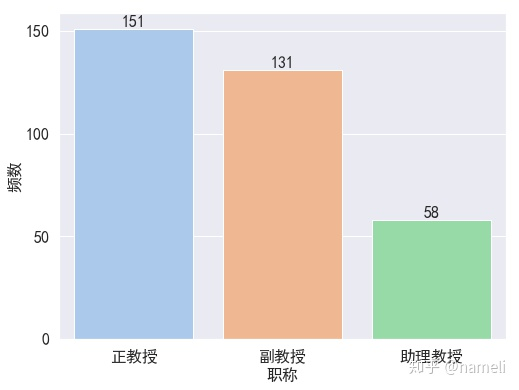图  1-1    不同职称人员频数      柱状图是针对离散型数据（比如职称），每根柱子代表一个类别（正教授、副教授、助理教授），柱子的高度就是这个类别的频数，也可以是百分比。    从上图来说明一个完整统计图应包含的注意事项：要有图表题，一般在图的下方，标题要简洁明了，比如图1-1的“不同职称人员频数”。报告中统计图要有标号。横轴和纵轴要标注清楚（横轴：职称， 纵轴：频数）。如有单位，还需注明。图的标题、横轴、纵轴等，出现的文字要统一和准确，不要一会儿中文，一会儿英文。写中文报告，就要标注中文。图的比例要协调，别太胖也别太瘦，别太高也别太矮。图的内容要正确、简明，避免出现不必要的标签、背景等。注意图的配色。不要用非常难看的配色！画完图要有适当的评论，尤其在报告里，这点非常重要。比如在图1-1中，可以写以下评述：         职称一共有三个水平（正教授、副教授和助理教授）。从图1-1可以看出，正教授人数最多（151人），其次是副教授（131人），人数最少的是助理教授（58人）。很多报告，常常是统计图从天而降，没有任何评述，这是非常糟糕的做法。要么不画图，既然画图，就要写评论。3  Python3代码建立DataFrames1 = pd.DataFrame({
'职称': {0:"正教授", 1:"副教授", 2:"助理教授"},
'频数': {0:151, 1:131, 2:58}
})2. 画图plt.rcParams['figure.figsize'] = (8.0, 6.0)   #调整图片大小

from matplotlib.font_manager import FontProperties   #显示中文，并指定字体
myfont=FontProperties(fname=r'C:WindowsFontssimhei.ttf',size=14)
sns.set(font=myfont.get_name())

ax = sns.barplot(x="职称", y="频数", data=s1, palette="pastel")   #seaborn画柱状图

plt.xticks(fontsize=16)          #设置x和y轴刻度值字体大小
plt.yticks(fontsize=16)

plt.yticks(np.arange(0, 151, 50))   #设置y轴标签

plt.xlabel("职称", fontsize=16)  #设置x轴和y轴标签字体大小
plt.ylabel("频数", fontsize=16)

x = np.arange(len(s1["职称"]))    #在柱状图上插入数值
y = np.array(list(s1["频数"]))
for a,b in zip(x,y):
plt.text(a, b+0.05, '%.0f' % b, ha='center', va= 'bottom',fontsize=16)

plt.show()4    参考文献王汉生《数据思维——从数据分析到商业价值》
展开全文• ﻿前言本文目标在于利用 快速画出符合自己要求的柱状图。最近在处理某一组成绩数据的时候，涉及了柱状图的画法，因此此处进行一下记录。加载库import matplotlib.pyplot as plt import matplotlib.font_manager as ...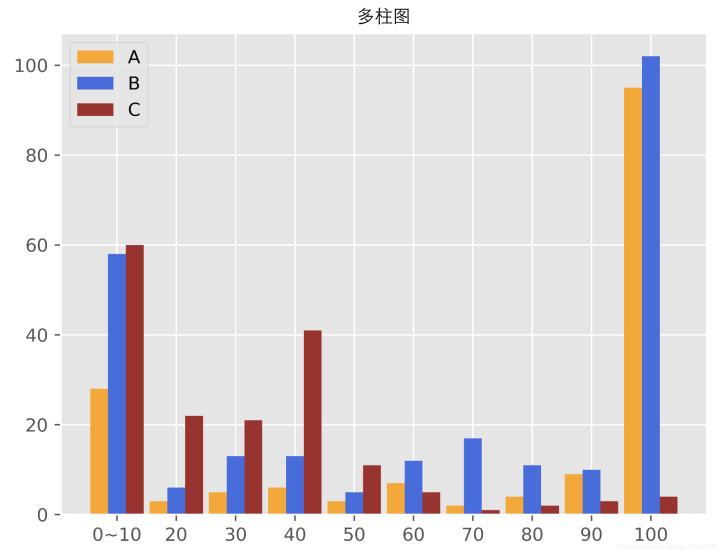﻿前言本文目标在于利用快速画出符合自己要求的柱状图。最近在处理某一组成绩数据的时候，涉及了柱状图的画法，因此此处进行一下记录。加载库import matplotlib.pyplot as plt
import matplotlib.font_manager as mfm
from matplotlib import style

style.use('ggplot')  # 加载'ggplot'风格

# 加载中文字体
font_path = "/System/Library/Fonts/STHeiti Light.ttc" # 本地字体链接
prop = mfm.FontProperties(fname=font_path)单一柱图其中表示分数在区间内的人数。total = [3, 5, 6, 7, 8, 6, 4, 3, 3, 22, 4, 8, 7, 13, 7, 7, 15, 10, 6, 52, 8, 2, 3, 26, 1, 1, 0, 2, 0, 3]如果直接对于上述数据执行下述代码，将得到如下结果。plt.bar(range(len(total)), total)
plt.title('单一柱图', fontproperties=prop)
plt.savefig("单一柱图.png", dpi=700, fontproperties=prop)
plt.show()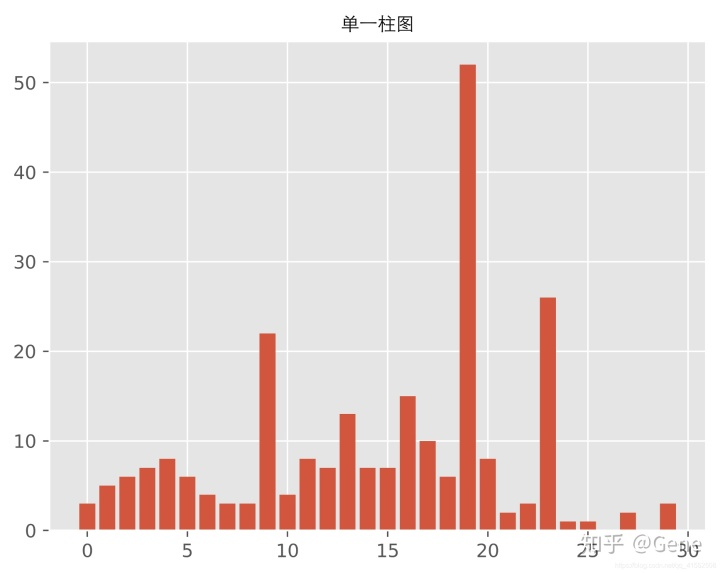不难发现，上述结果的效果不好。首先第一点，分数段是，而此处显示的是后的数值。其次，最后一根柱子没有数据显示，但其实应该显示的数据，因此我们需要对此进行改进。自定义横坐标因此我们选择自定义横坐标的方式来进行效果改进，具体代码如下。x_labels = []

for item in range(0, 300, 10):
x = item + 10
if x == 10:
x_labels.append("{}~{}".format(0, 10))
elif x % 50 == 0:
x_labels.append("{}".format(x))
else:
x_labels.append(None)

x = range(len(total))
plt.bar(x, total)
plt.title('单一柱图', fontproperties=prop)
plt.xticks(x, x_labels)
plt.savefig("单一柱图.png", dpi=700, fontproperties=prop)
plt.show()我们在个区间中仅选取了一部分进行标注，以及令第一个区间以的方式进行显示。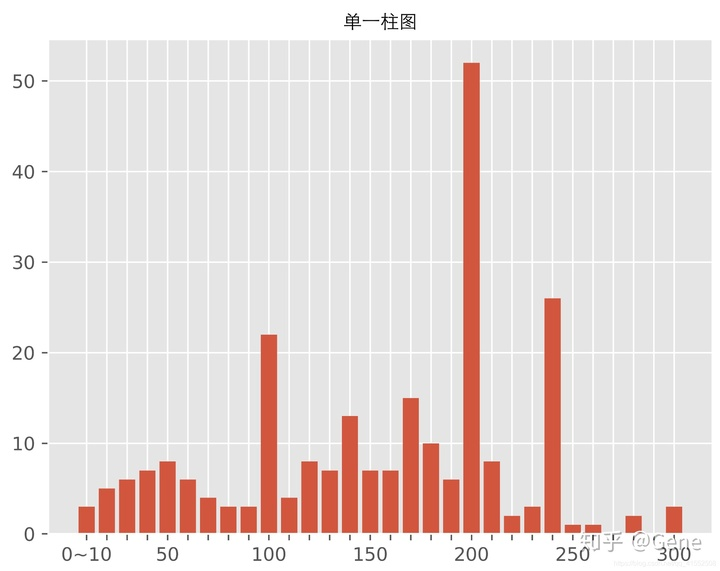多柱图在展示完单一柱图之后，我们进入多柱同时显示的代码内容。首先是数据内容的展示，表示第题得分在范围内的人数。A = [[28, 3, 5, 6, 3, 7, 2, 4, 9, 95],
[58, 6, 13, 13, 5, 12, 17, 11, 10, 102],
[60, 22, 21, 41, 11, 5, 1, 2, 3, 4]]然后我们执行下述代码即可得到下述多柱图。# 生成横坐标
x_labels = []
for item in range(0, 100, 10):
x = item + 10
if x == 10:
x_labels.append("{}~{}".format(0, 10))
else:
x_labels.append("{}".format(x))
# 生成横坐标范围
x = np.arange(10)
# 生成多柱图
plt.bar(x + 0.00, A, color='orange', width=0.3, label="A")
plt.bar(x + 0.30, A, color='royalblue', width=0.3, label="B")
plt.bar(x + 0.60, A, color='brown', width=0.3, label="C")
# 图片名称
plt.title('多柱图', fontproperties=prop)
# 横坐标绑定
plt.xticks(x + 0.30, x_labels)
# 生成图片
plt.legend(loc="best")
plt.savefig("多柱图.png", dpi=700, fontproperties=prop)
plt.show()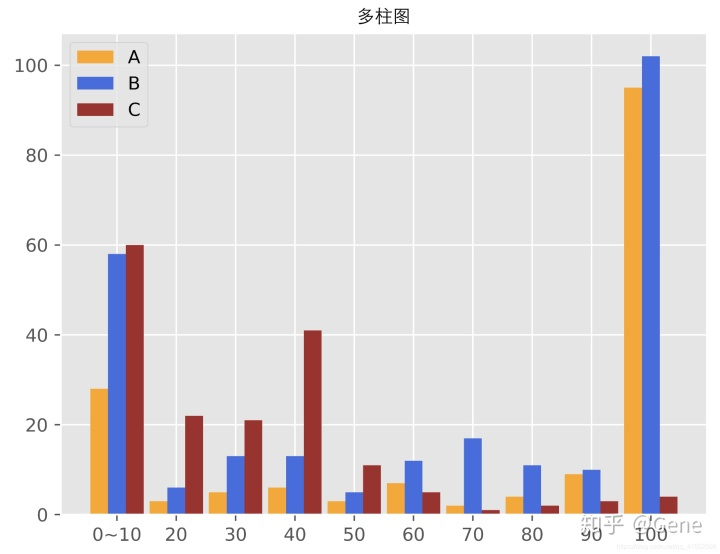后记至此，Python 柱状图 的基础操作就介绍完毕了，本文也算是对于上一篇 《Python 画图基础操作详解》 文章的一个补充，不过仍然还有很多其他类型图的画法没有介绍，感兴趣的朋友可以继续深入研究！最后祝大家画图快乐，在 Python 的画图之路上更进一步！   
展开全文• 今天为大家带来极坐标柱状图，一起来学习下吧~柱状图是一种以长方形长度为变量的统计报告图，由一系列高度不等的纵向条纹表示数据分布的情况。极坐标柱状图则是将柱形条显示在极坐标中，其不仅保留了直角坐标系中...
之前我们讲过极坐标散点图，不知道大家还有印象没？今天为大家带来极坐标柱状图，一起来学习下吧~柱状图是一种以长方形长度为变量的统计报告图，由一系列高度不等的纵向条纹表示数据分布的情况。极坐标柱状图则是将柱形条显示在极坐标中，其不仅保留了直角坐标系中数据变化趋势的优点，而且图形表达形式也更加美观。N = 20theta = np.linspace(0.0, 2 * np.pi, N, endpoint=False)# 从0到2pi生成均匀间隔的20个数，endpoint为Flase表示不包含末尾数字2pi，默认为True，这里指的是角度R = 10 * np.random.rand(N) # 随机生成20个半径width = np.pi / 8 * np.random.rand(N) # 线的宽度ax = plt.subplot(111, projection = 'polar') # 极坐标图'polar'bars = ax.bar(theta,R, width = width, bottom = 0.0) # 绘制柱子# 利用循环设置每个柱子的颜色、透明度for r, bar in zip(radii, bars): bar.set_facecolor(plt.cm.viridis(r / 10.)) # 设置颜色 bar.set_alpha(0.5) # 设置透明度plt.show()所作图形如下所示，可以看到，图形可以很清楚地传达数据的大小，一如你在柱状图(条形图)上看到的，你还可以尝试在圆外添加标签，使得柱条与标签一一对应起来(不会的话请继续阅读下文极坐标雷达图的绘制)。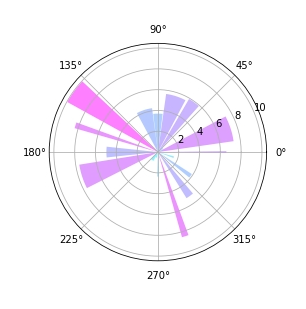以上就是python画极坐标柱状图的方法。更多Python学习推荐:JQ教程网Python大全。
展开全文• Plotly的官方网站为：https://plot.ly/python量化的关键是金融数据可视化，无论是传统的K线，还是现在的互动策略分析，都需要大量的可视化图表。具体到编程代码，就是各种python绘图模块库，传统的python绘图模块...
• 推荐图书：《Python可以这样学》，ISBN：9787302456469，董付国，清华大学出版社，第9次印刷图书详情(京东)：董付国老师所有图书均提供配套教学资源。==============功能描述：给定信号，对其进行傅里叶变换然后再...
• 一、背景重绘岩石电阻率分布图二、基本原理绘制一个横向的堆积柱状图，把地下的柱状图填充为白色，作为占位。三、数据整理通过上网查找到一些常见岩石的电阻率分布范围将数据重新在 Excel中编辑，保存为 new 1.csv ...
• R语言的ggplot2绘图能力超强，python虽有matplotlib，但是语法臃肿，使用复杂，入门极难，seaborn的出现稍微改善了matplotlib代码量问题，但是定制化程度依然需要借助matplotlib，使用难度依然很大。好消息是python...
• Seaborn是Python中的一个库，主要用于生成统计图形。Seaborn是构建在matplotlib之上的数据可视化库，与Python中的pandas数据结构紧密集成。可视化是Seaborn的核心部分，可以帮助探索和理解数据。 要了解Seaborn，就...
• 南丁格尔玫瑰，又被称为极区，是一种比较高级的图形。其百度百科解释如下：南丁格尔玫瑰是弗...绘制南丁格尔玫瑰，有很多种方法，比如我们可以使用Python，可以使用R语言，甚至于说，我们可以使用Excel来...
• 对商业智能BI、大数据分析挖掘、机器学习，python，R等数据领域感兴趣的同学加微信：tstoutiao，邀请你进入数据爱好者交流群，数据爱好者们都在这儿。今天这篇博文为大家分享如何用ggplot2绘制漂亮的直方(如封面所...
• #creat a dataset(生成数据)specieconditionvaluedflibrary(ggplot2)#分组柱形p1p1+theme(legend.position = "none")#去掉右侧图例p1+scale_fill_manual(values=c("blue","red","green"))#自定义填充色Rplot09....
• 说到python可视化库，大家可能第一时间想到matplotlib。matplotlib算是python比较底层的可视化库，可定制性强、图表资源丰富、简单易用、并且达到出版质量级别。除了matplotlib还有其它几十种优秀的可视化库哦！这篇...
• 生信分析中经常会得到一些基因，然后做GO富集分析，达到对基因进行注释和分类的目的。...library(ggplot2)data 二 对上述GO结果绘制基础bar参照之前ggplot2使用方法，更改geom即可绘制简单的bar，按照G...
• 导读ggplot2函数包ggplot函数geom_bar参数画柱形。利用apply(df, FUN=sum)求和利用df[order(df\$sum, decreasing=T),]倒序利用scale_x_discrete(limits=factor())设置横轴排序一、输入数据set.seed(1995)# 随机种子...
• 大致浏览一下Python库索引，你将会看到几乎每个数据可视化需要的库，从用于眼动研究的GazeParser到用于神经网络训练的实时可视化的pastalog。虽然这些库中有很多库只能完成一些特定任务，但有些库可以应用于任何领域...
• 在excel中给一个数据表做相应的图表时怎么把纵轴的单位变大 在数据上选设置坐标轴格式,修改参数即可. 如何在excel中将数据作为表格的横纵坐标? Excel中，可过制定横纵坐方式，将数据作为图表的横纵坐标。 软件...
• image.png之前有读者在公众号留言问开头的应该如何画？我第一眼看到这个简单的理解为了堆积柱形，加折线。所以基本的想法就是构造两份数据来画这个，第一份用来画堆积柱形，第二份用来画折线。但是...
• 我们粉丝里面有很多小伙伴喜欢玩数据分析，粗略估计有几千人。数据分析离不开数据...神器PlotlyPlotly 是一款用来做数据分析和可视化的在线平台，功能非常强大,可以在线绘制很多图形比如条形、散点、饼图、直...
• I'm trying to make a histogram of a simple list of numbers in python using ipython notebook and ggplot for python. Using pylab, it's easy enough, but I cannot get ggplot to work.I'm using this code (b...
• 今天，我们将简要介绍...Matplotlib两个直方（matplotlib）matplotlib是Python数据可视化库中的泰斗。尽管它已有十多年的历史，但它仍是Python使用者最广泛使用的绘画库。其设计与20世纪80年代开发的一种专有编程...
• (点击上方快速关注并设置为星标，一起学Python)译者：欧剃 编辑：肉松 来源：...Python 绘图库Plotly，教你如何用超简单...
• 而在绘制柱状图时，对于x时分类变量的情况且设置y参数时，用pn.geom_bar()需要设置geom_bar的参数为 stat = "identity"，否则会报错PlotnineError，另一个plotnine中绘制柱状图的pn.geom_col()则不需要额外设置...
• 作者：Will Koehrsen，译者：欧剃，编辑：肉松原文：https://towardsdatascience.com/the-next-level-of-data-visualization-in-python-dd6e99039d5e来自...介绍的是功能强大的开源Python 绘图库Plotly，教你如何...
• 获取慢日志统计柱状图默认接口请求频率限制：5次秒。 注意：本接口支持金融区地域。 由于金融区和非金融区是隔离不互通的，因此当公共参数 region 为金融区地域（例如 ap-shanghai-fsi）时，需要同时指定带金融区...
• 相对R，python在图形可视化相对弱化一些，考虑python其他强大功能，逐步学习python实现图的一些代码，学习DataCharm公众号的柱状图后，实现效果如下：实现代码如下：import numpy as npimport matplotlib.pyplot as ...
• 本文实例为大家分享了python使用Plotly绘图工具绘制柱状图的具体代码，供大家参考，具体内容如下使用Plotly绘制基本的柱状图，需要用到的函数是graph_objs 中 Bar函数通过参数，可以设置柱状图的样式。通过barmod...
• #导入相应的包 import pandas as pd import matplotlib.pyplot as plt ...os.chdir('E://xh/python代码')#设置路径 df=pd.read_excel('Mean_std.xls',sheet_name='Sheet3') 数据筛选 y1=df['Mean'].loc[...
• 象形柱图是可以设置各种具象图形...下面给大家介绍一下常见象形柱状图的画法：一、基本象形柱状图基本象形柱状图较普通柱状图，就是把图形用具体图像表示，pyecharts提供的标记类型包括 'circle', 'rect', 'roundRe...
• Python 使用 matplotlib 画图是非常方便的，之前的文章记录了《Python 使用 matplotlib 画折线图教程》，今天就再次记录一下使用 matplotlib 画柱状图的教程。一般来说，也就折线图和柱状图这两种图比较常见，所以......

# 柱状图pythonpython 订阅